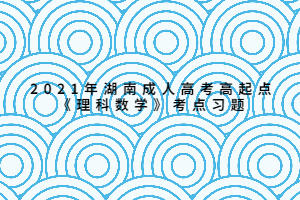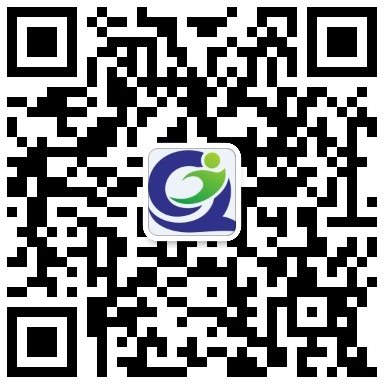• 欢迎访问

# 上海成考网

！本网站为民间交流网站，主要为上海成人高考考生提供报考指导服务，网站信息仅供学习交流使用，官方信息以上海教育考试院www.shmeea.edu.cn为准。+关注# 2021年湖南成人高考高起点《理科数学》考点习题：直线与简易逻辑

【摘要】【导读】在成人高考中数学是很多考生头疼的科目，但其实数学是有技巧的，他的解题思路，分析题目的思路都很重要，只要你摸透了，就知道其实数学也没有那么难。以下是上海成人...

【导读】在成人高考中数学是很多考生头疼的科目，但其实数学是有技巧的，他的解题思路，分析题目的思路都很重要，只要你摸透了，就知道其实数学也没有那么难。以下是上海成人高考网整理2021年湖南成人高考高起点《理科数学》考点习题，供考生练习，希望对各位考生有所帮助。1[.单选题]分别和两条异面直线AB、CD同时相交的两条直线AC、BD()。

A.相交

B.平行

C.是异面直线

D.垂直

2[.单选题]两条直线是异面直线的充分条件是这两条直线()。

A.分别在两个平面内

B.是分别在两个相交平面内的不相交的直线

C.是分别在两个相交平面内的不平行的直线

D.分别在两个相交平面内，其中一条与这两个平面的交线相交于一点，而另一条不过这个点

3[.单选题]抛物线y2=2px上任意一点与焦点连线中点的轨迹方程是()()。

A.y2=2p(x-p/2)

B.y2=2p(x-p/4)

C.y2=p(x-p/2)

D.y2=p(x-p/4)

4[.单选题]平面内有12个点，任何三点不在同一直线上，以每三点为顶点画一个三角形，一共可画三角形()。

A.36个

B.220个

C.660个

D.1320个

5[.单选题]若点(4，a)到直线4x-3y-1=0的距离不大于3，则a的取值范围是()。

A.(0，10)

B.[0，10]

C.(10，30)

D.(-10，10)

6[.单选题]曲线y=x3+2x-1在点M(1，2)处的切线方程是()。

A.5x-y-3=0

B.x-5y-3=0

C.5x+y-3=0

D.x+5y-3=0

7[.单选题]以抛物线y2=8x的焦点为圆心，且与此抛物线的准线相切的圆的方程是()。

A.(x+2)2+y2=16

B.(x+2)2+y2=4

C.(x-2)2+y2=16

D.(x-2)2+y2=4

8[.单选题]圆x2+y2=25上的点到直线5x+12y-169=0的距离的最小值是()。

A.9

B.8

C.7

D.6

9[.单选题]设F1和F2为双曲线x2/4-y2=1的两焦点，点p在双曲线上，则||PF1|—|PF2||=()。

A.4

B.2

C.1

D.

1/4

10[.单选题]抛物线y2=4x上一点P到焦点F的距离是10，则点P坐标是()。

A.(9，6)

B.(9，±6)

C.(6，9)

D.(±6，9)

11[.单选题]下列四个命题中正确的是()。

①已知a，b，c三条直线，其中a，b异面，a//c，则b，c异面。

②若a与b异面，b与c异面，则a与c异面。

③过平面外一点与平面内一点的直线，和平面内不经过该点的直线是异面直线。

④不同在任何一个平面内的两条直线叫异面直线。

A.③④

B.②③④

C.①②③④

D.①②

12[.单选题]过直线3x+2y+1=0与2x-3y+5=0的交点，且垂直于直线L：6x-2y+5=0的直线方程是()。

A.x-3y-2=0

B.x+3y-2=0

C.x-3y+2=0

D.x+3y+2=0

13[.单选题]抛物线y=ax2的准线方程是y=2,则a=()。

A.1/8

B.-1/8

C.8

D.-8

14[.单选题]已知圆的方程为x2+y2-2x+4y+1=0，则圆上一点到直线3x+4y-10=0的最大距离为()。

A.6

B.5

C.4

D.3

15[.单选题]在△ABC中，已知AB=5，AC=3，∠A=120°，则BC长为()。

A.7

B.6

C.√20

D.√19

16[.单选题]抛物线x=-1/2y2的准线方程是()

A.x=1

B.y=1

C.x=-1

D.y=-1### 热门文章